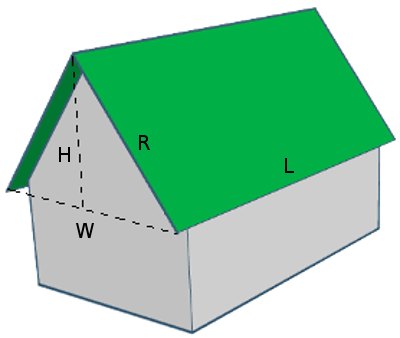# How to calculate the roof area from for a Gabled roofIn this article we will talk about how to calculate the roof area for a gabled roof.
The most important value we have to calculate here is the Rafter length (R).
Once we have R, we can easily detremine the surface of the roof.
To calculate R, we need to know the Length of the roof (L), the width of the roof (W) and the Height (H).
If we have this values, we can determine the R as the hypotenuse of the triangle H, W/2, R - by using the pythagorean theorem.
Roof Length (L):
%
Roof Width (W):
%
Roof Rise or Height (H):
%
Using the pythagorean theorem, we can calculate R
R² = H² + (W/2)²
R² = ² + ( / 2)²
R² = +
R² =
R =
Now that we have R, we can calculate the surface of one slope (S1) - this is a parallelogram and its aria is R (roof rafter) x L (roof length)
S1 = R x L
S1 = x
S1 =
And Total surface of a gabled roof - is S1 x 2 (as we have to identical slopes)
Total Surface = x 2
Total Surface =